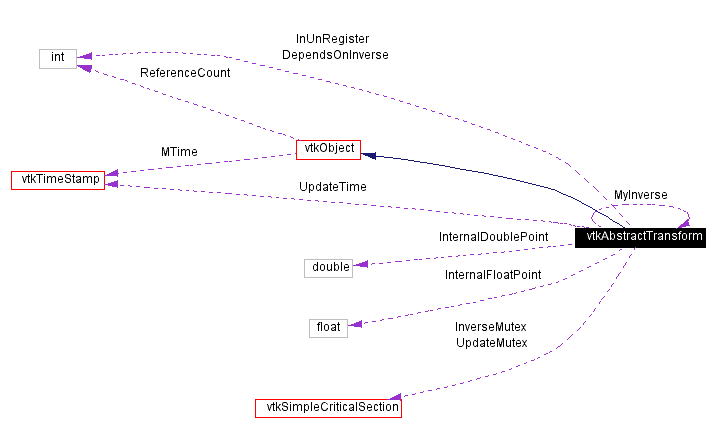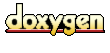Main Page   Class Hierarchy   Alphabetical List   Compound List   File List   Compound Members   File Members   Related Pages

# vtkAbstractTransform Class Reference

superclass for all geometric transformations. More...

`#include <vtkAbstractTransform.h>`

Inheritance diagram for vtkAbstractTransform:[legend]
Collaboration diagram for vtkAbstractTransform:[legend]
List of all members.

## Public Methods

virtual const char * GetClassName ()
virtual int IsA (const char *type)
void PrintSelf (ostream &os, vtkIndent indent)
double * TransformNormalAtPoint (const double point, const double normal)
double * TransformVectorAtPoint (const double point, const double vector)
virtual void TransformPoints (vtkPoints *inPts, vtkPoints *outPts)
vtkAbstractTransform * GetInverse ()
void SetInverse (vtkAbstractTransform *transform)
virtual void Inverse ()=0
void DeepCopy (vtkAbstractTransform *)
void Update ()
virtual vtkAbstractTransform * MakeTransform ()=0
virtual int CircuitCheck (vtkAbstractTransform *transform)
unsigned long GetMTime ()
void UnRegister (vtkObject *O)
void TransformPoint (const float in, float out)
void TransformPoint (const double in, double out)
double * TransformPoint (double x, double y, double z)
double * TransformPoint (const double point)
float * TransformFloatPoint (float x, float y, float z)
float * TransformFloatPoint (const float point)
double * TransformDoublePoint (double x, double y, double z)
double * TransformDoublePoint (const double point)
void TransformNormalAtPoint (const float point, const float in, float out)
void TransformNormalAtPoint (const double point, const double in, double out)
double * TransformDoubleNormalAtPoint (const double point, const double normal)
float * TransformFloatNormalAtPoint (const float point, const float normal)
void TransformVectorAtPoint (const float point, const float in, float out)
void TransformVectorAtPoint (const double point, const double in, double out)
double * TransformDoubleVectorAtPoint (const double point, const double vector)
float * TransformFloatVectorAtPoint (const float point, const float vector)
virtual void TransformPointsNormalsVectors (vtkPoints *inPts, vtkPoints *outPts, vtkDataArray *inNms, vtkDataArray *outNms, vtkDataArray *inVrs, vtkDataArray *outVrs)
virtual void InternalTransformPoint (const float in, float out)=0
virtual void InternalTransformPoint (const double in, double out)=0
virtual void InternalTransformDerivative (const float in, float out, float derivative)=0
virtual void InternalTransformDerivative (const double in, double out, double derivative)=0
void Identity ()

## Static Public Methods

int IsTypeOf (const char *type)
vtkAbstractTransform * SafeDownCast (vtkObject *o)

## Protected Methods

vtkAbstractTransform ()
~vtkAbstractTransform ()
virtual void InternalUpdate ()
virtual void InternalDeepCopy (vtkAbstractTransform *)

## Protected Attributes

float InternalFloatPoint 
double InternalDoublePoint 

## Detailed Description

superclass for all geometric transformations.

Date:
2001/11/30 19:14:13
Revision:
1.16
Thanks:
Thanks to David G. Gobbi who developed this class.
vtkAbstractTransform is the superclass for all VTK geometric transformations. The VTK transform hierarchy is split into two major branches: warp transformations and homogeneous (including linear) transformations. The latter can be represented in terms of a 4x4 transformation matrix, the former cannot.

Transformations can be pipelined through two mechanisms:

1) GetInverse() returns the pipelined inverse of a transformation i.e. if you modify the original transform, any transform previously returned by the GetInverse() method will automatically update itself according to the change.

2) You can do pipelined concatenation of transformations through the vtkGeneralTransform class, the vtkPerspectiveTransform class, or the vtkTransform class.

See also:
vtkGeneralTransform vtkWarpTransform vtkHomogeneousTransform vtkLinearTransform vtkIdentityTransform vtkTransformPolyDataFilter vtkTransformFilter vtkImageReslice vtkImplicitFunction

Definition at line 77 of file vtkAbstractTransform.h.

## Constructor & Destructor Documentation

 vtkAbstractTransform::vtkAbstractTransform ( ) ` [protected]`

 vtkAbstractTransform::~vtkAbstractTransform ( ) ` [protected]`

## Member Function Documentation

 virtual const char* vtkAbstractTransform::GetClassName ( ) ` [virtual]`
 Return the class name as a string. This method is defined in all subclasses of vtkObject with the vtkTypeMacro found in vtkSetGet.h. Reimplemented from vtkObject.

 int vtkAbstractTransform::IsTypeOf ( const char * type ) ` [static]`
 Return 1 if this class type is the same type of (or a subclass of) the named class. Returns 0 otherwise. This method works in combination with vtkTypeMacro found in vtkSetGet.h. Reimplemented from vtkObject.

 virtual int vtkAbstractTransform::IsA ( const char * type ) ` [virtual]`
 Return 1 if this class is the same type of (or a subclass of) the named class. Returns 0 otherwise. This method works in combination with vtkTypeMacro found in vtkSetGet.h. Reimplemented from vtkObject.

 vtkAbstractTransform* vtkAbstractTransform::SafeDownCast ( vtkObject * o ) ` [static]`
 Will cast the supplied object to vtkObject* is this is a safe operation (i.e., a safe downcast); otherwise NULL is returned. This method is defined in all subclasses of vtkObject with the vtkTypeMacro found in vtkSetGet.h. Reimplemented from vtkObject.

 void vtkAbstractTransform::PrintSelf ( ostream & os, vtkIndent indent ) ` [virtual]`
 Methods invoked by print to print information about the object including superclasses. Typically not called by the user (use Print() instead) but used in the hierarchical print process to combine the output of several classes. Reimplemented from vtkObject.

 void vtkAbstractTransform::TransformPoint ( const float in, float out ) ` [inline]`
 Apply the transformation to a coordinate. You can use the same array to store both the input and output point. Definition at line 87 of file vtkAbstractTransform.h.

 void vtkAbstractTransform::TransformPoint ( const double in, double out ) ` [inline]`
 Apply the transformation to a double-precision coordinate. You can use the same array to store both the input and output point. Definition at line 94 of file vtkAbstractTransform.h.

 double* vtkAbstractTransform::TransformPoint ( double x, double y, double z ) ` [inline]`
 Apply the transformation to a double-precision coordinate. Use this if you are programming in Python, tcl or Java. Definition at line 101 of file vtkAbstractTransform.h.

 double* vtkAbstractTransform::TransformPoint ( const double point ) ` [inline]`
 Apply the transformation to a double-precision coordinate. Use this if you are programming in Python, tcl or Java. Definition at line 103 of file vtkAbstractTransform.h.

 float* vtkAbstractTransform::TransformFloatPoint ( float x, float y, float z ) ` [inline]`
 Apply the transformation to an (x,y,z) coordinate. Use this if you are programming in Python, tcl or Java. Definition at line 110 of file vtkAbstractTransform.h.

 float* vtkAbstractTransform::TransformFloatPoint ( const float point ) ` [inline]`
 Apply the transformation to an (x,y,z) coordinate. Use this if you are programming in Python, tcl or Java. Definition at line 116 of file vtkAbstractTransform.h.

 double* vtkAbstractTransform::TransformDoublePoint ( double x, double y, double z ) ` [inline]`
 Apply the transformation to a double-precision (x,y,z) coordinate. Use this if you are programming in Python, tcl or Java. Definition at line 123 of file vtkAbstractTransform.h.

 double* vtkAbstractTransform::TransformDoublePoint ( const double point ) ` [inline]`
 Apply the transformation to a double-precision (x,y,z) coordinate. Use this if you are programming in Python, tcl or Java. Definition at line 129 of file vtkAbstractTransform.h.

 void vtkAbstractTransform::TransformNormalAtPoint ( const float point, const float in, float out )
 Apply the transformation to a normal at the specified vertex. If the transformation is a vtkLinearTransform, you can use TransformNormal() instead.

 void vtkAbstractTransform::TransformNormalAtPoint ( const double point, const double in, double out )
 Apply the transformation to a normal at the specified vertex. If the transformation is a vtkLinearTransform, you can use TransformNormal() instead.

 double* vtkAbstractTransform::TransformNormalAtPoint ( const double point, const double normal ) ` [inline]`
 Definition at line 143 of file vtkAbstractTransform.h.

 double* vtkAbstractTransform::TransformDoubleNormalAtPoint ( const double point, const double normal ) ` [inline]`
 Apply the transformation to a double-precision normal at the specified vertex. If the transformation is a vtkLinearTransform, you can use TransformDoubleNormal() instead. Definition at line 152 of file vtkAbstractTransform.h.

 float* vtkAbstractTransform::TransformFloatNormalAtPoint ( const float point, const float normal ) ` [inline]`
 Apply the transformation to a single-precision normal at the specified vertex. If the transformation is a vtkLinearTransform, you can use TransformFloatNormal() instead. Definition at line 162 of file vtkAbstractTransform.h.

 void vtkAbstractTransform::TransformVectorAtPoint ( const float point, const float in, float out )
 Apply the transformation to a vector at the specified vertex. If the transformation is a vtkLinearTransform, you can use TransformVector() instead.

 void vtkAbstractTransform::TransformVectorAtPoint ( const double point, const double in, double out )
 Apply the transformation to a vector at the specified vertex. If the transformation is a vtkLinearTransform, you can use TransformVector() instead.

 double* vtkAbstractTransform::TransformVectorAtPoint ( const double point, const double vector ) ` [inline]`
 Definition at line 178 of file vtkAbstractTransform.h.

 double* vtkAbstractTransform::TransformDoubleVectorAtPoint ( const double point, const double vector ) ` [inline]`
 Apply the transformation to a double-precision vector at the specified vertex. If the transformation is a vtkLinearTransform, you can use TransformDoubleVector() instead. Definition at line 187 of file vtkAbstractTransform.h.

 float* vtkAbstractTransform::TransformFloatVectorAtPoint ( const float point, const float vector ) ` [inline]`
 Apply the transformation to a single-precision vector at the specified vertex. If the transformation is a vtkLinearTransform, you can use TransformFloatVector() instead. Definition at line 197 of file vtkAbstractTransform.h.

 virtual void vtkAbstractTransform::TransformPoints ( vtkPoints * inPts, vtkPoints * outPts ) ` [virtual]`
 Apply the transformation to a series of points, and append the results to outPts. Reimplemented in vtkHomogeneousTransform, vtkIdentityTransform, and vtkLinearTransform.

 virtual void vtkAbstractTransform::TransformPointsNormalsVectors ( vtkPoints * inPts, vtkPoints * outPts, vtkDataArray * inNms, vtkDataArray * outNms, vtkDataArray * inVrs, vtkDataArray * outVrs ) ` [virtual]`
 Apply the transformation to a combination of points, normals and vectors. Reimplemented in vtkHomogeneousTransform, vtkIdentityTransform, and vtkLinearTransform.

 vtkAbstractTransform* vtkAbstractTransform::GetInverse ( )
 Get the inverse of this transform. If you modify this transform, the returned inverse transform will automatically update. If you want the inverse of a vtkTransform, you might want to use GetLinearInverse() instead which will type cast the result from vtkAbstractTransform to vtkLinearTransform. Reimplemented in vtkTransform.

 void vtkAbstractTransform::SetInverse ( vtkAbstractTransform * transform )
 Set a transformation that this transform will be the inverse of. This transform will automatically update to agree with the inverse transform that you set.

 virtual void vtkAbstractTransform::Inverse ( ) ` [pure virtual]`
 Invert the transformation.

 void vtkAbstractTransform::DeepCopy ( vtkAbstractTransform * )
 Copy this transform from another of the same type.

 void vtkAbstractTransform::Update ( )
 Update the transform to account for any changes which have been made. You do not have to call this method yourself, it is called automatically whenever the transform needs an update. Referenced by vtkHomogeneousTransform::GetMatrix(), vtkLinearTransform::TransformNormal(), and vtkLinearTransform::TransformVector().

 virtual void vtkAbstractTransform::InternalTransformPoint ( const float in, float out ) ` [pure virtual]`
 This will calculate the transformation without calling Update. Meant for use only within other VTK classes. Reimplemented in vtkGeneralTransform, vtkHomogeneousTransform, vtkIdentityTransform, vtkLinearTransform, and vtkWarpTransform.

 virtual void vtkAbstractTransform::InternalTransformPoint ( const double in, double out ) ` [pure virtual]`
 This will calculate the transformation without calling Update. Meant for use only within other VTK classes. Reimplemented in vtkGeneralTransform, vtkHomogeneousTransform, vtkIdentityTransform, vtkLinearTransform, and vtkWarpTransform.

 virtual void vtkAbstractTransform::InternalTransformDerivative ( const float in, float out, float derivative ) ` [pure virtual]`
 This will transform a point and, at the same time, calculate a 3x3 Jacobian matrix that provides the partial derivatives of the transformation at that point. This method does not call Update. Meant for use only within other VTK classes. Reimplemented in vtkGeneralTransform, vtkHomogeneousTransform, vtkIdentityTransform, vtkLinearTransform, and vtkWarpTransform.

 virtual void vtkAbstractTransform::InternalTransformDerivative ( const double in, double out, double derivative ) ` [pure virtual]`
 This will transform a point and, at the same time, calculate a 3x3 Jacobian matrix that provides the partial derivatives of the transformation at that point. This method does not call Update. Meant for use only within other VTK classes. Reimplemented in vtkGeneralTransform, vtkHomogeneousTransform, vtkIdentityTransform, vtkLinearTransform, and vtkWarpTransform.

 virtual vtkAbstractTransform* vtkAbstractTransform::MakeTransform ( ) ` [pure virtual]`
 Make another transform of the same type.

 virtual int vtkAbstractTransform::CircuitCheck ( vtkAbstractTransform * transform ) ` [virtual]`
 Check for self-reference. Will return true if concatenating with the specified transform, setting it to be our inverse, or setting it to be our input will create a circular reference. CircuitCheck is automatically called by SetInput(), SetInverse(), and Concatenate(vtkXTransform *). Avoid using this function, it is experimental. Reimplemented in vtkGeneralTransform, vtkPerspectiveTransform, and vtkTransform.

 unsigned long vtkAbstractTransform::GetMTime ( ) ` [virtual]`
 Override GetMTime necessary because of inverse transforms. Reimplemented from vtkObject.

 void vtkAbstractTransform::UnRegister ( vtkObject * O ) ` [virtual]`
 Needs a special UnRegister() implementation to avoid circular references. Reimplemented from vtkObject.

 void vtkAbstractTransform::Identity ( ) ` [inline]`
 This method is deprecated in the base class. It is still valid to use it on many of the specialized classes. Reimplemented in vtkGeneralTransform, vtkPerspectiveTransform, and vtkTransform. Definition at line 280 of file vtkAbstractTransform.h. Referenced by vtkTransform::SetMatrix(), and vtkPerspectiveTransform::SetMatrix().

 virtual void vtkAbstractTransform::InternalUpdate ( ) ` [inline, protected, virtual]`
 Perform any subclass-specific Update. Definition at line 289 of file vtkAbstractTransform.h.

 virtual void vtkAbstractTransform::InternalDeepCopy ( vtkAbstractTransform * t ) ` [inline, protected, virtual]`
 Perform any subclass-specific DeepCopy. Definition at line 292 of file vtkAbstractTransform.h.

## Member Data Documentation

 float vtkAbstractTransform::InternalFloatPoint` [protected]`
 Definition at line 294 of file vtkAbstractTransform.h.

 double vtkAbstractTransform::InternalDoublePoint` [protected]`
 Definition at line 295 of file vtkAbstractTransform.h.

The documentation for this class was generated from the following file:

Generated on Thu Mar 28 14:27:12 2002 for VTK by1.2.11.1 written by Dimitri van Heesch, © 1997-2001